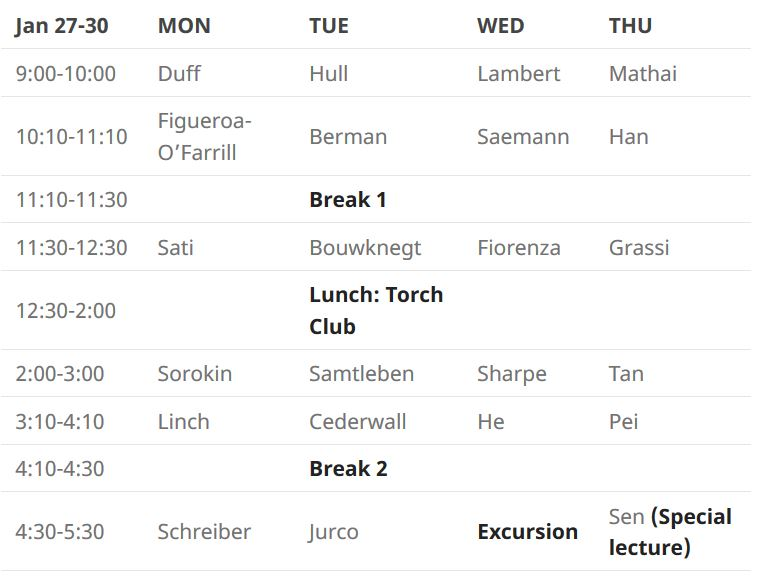# nLab M-Theory and Mathematics

M-Theory and Mathematics 2020

mtheorymath.org

A conference series on M-theory and mathematics.

These workshops bring together experts on the mathematical aspects of M-theory, the main candidate for a theory of everything, with implications to string theory and quantum field theory and interactions with geometry and algebraic topology.

## M-Theory and Mathematics 2020Confirmed speakers:

Abstracts, Slides and Talk notes:

• Bianca Cerchiai

Supergravity in a pencil

Abstract: In the spirit of the gauge-gravity correspondence, we derive a 2+1 dimensional model with “unconventional” supersymmetry at the boundary of a 4-dimensional Anti de Sitter N-extended supergravity, which in the case N=2 reproduces the AVZ model [P.D. Alvarez, M. Valenzuela, J. Zanelli, JHEP 1204 (2012) 058, arXiv:1109.3944 hep-th]. The extended supersymmetry of the boundary model is instrumental to describe the electronic properties of graphene, in particular at the two Dirac points. The two valleys correspond to the two independent sectors of the OSp(p|2)×OSp(q|2) boundary model in the p=q case, which are related by a parity transformation. The Semenoff and the Haldane masses entering the corresponding Dirac equations for the graphene pseudoparticles are identified with supergravity torsion parameters.

• Universes as Bigdata

Abstract: We review how historically the problem of string phenomenology lead theoretical physics first to algebraic/differential geometry, and then to computational geometry, and now to data science and AI. With the concrete playground of the Calabi-Yau landscape, accumulated by the collaboration of physicists, mathematicians and computer scientists over the last 4 decades, we show how the latest techniques in machine-learning can help explore problems of physical and mathematical interest.

• Lagrangians with (2,0) Supersymmetry

Abstract: We will discuss free and interacting six-dimensional actions which admit $(2,0)$ supersymmetry including their application to abelian and non-abelian M5-branes.

more to appear here…

Related events:

category: reference

Last revised on November 22, 2020 at 11:00:02. See the history of this page for a list of all contributions to it.